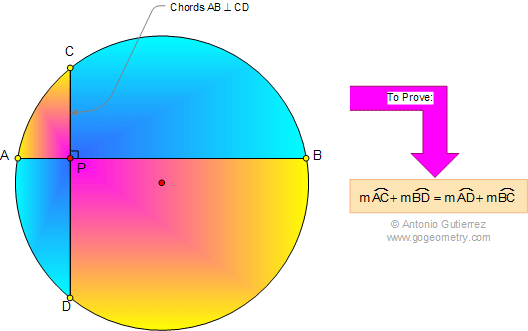# Geometry Problem 648: Archimedes' Book of Lemmas: Proposition 9, Perpendicular chords, Arcs

If two chords of a circle intersect at right angles, then the sum of the opposite intercepted arcs are equals. That is, if chords AB and CD are perpendiculars at P, then arc AC + arc BD = arc AD + arc BC.## Books of Lemmas Index